### O.C. and S.C. Tests on Single Phase Transformer

1. O.C. and S.C. Tests on Single Phase Transformer
The efficiency and regulation of a transformer on any load condition and at any power factor condition can be predetermined by indirect loading method. In this method, the actual load is not used on transformer. But the equivalent circuit parameters of a transformer are determined by conducting two tests on a transformer which are,
1. Open circuit test (O.C Test)
2. Short circuit test (S.C.Test)
The parameters calculated from these test results are effective in determining the regulation and efficiency of a transformer at any load and power factor condition, without actually loading the transformer. The advantage of this method is that without much power loss the tests can be performed and results can be obtained. Let us discuss in detail how to perform these tests and how to use the results to calculate equivalent circuit parameters.
1.1 Open Circuit Test (O.C. Test)
The experimental circuit to conduct O.C test is shown in the Fig. 1.Fig 1. Experimental circuit for O.C. test

The transformer primary is connected to a.c. supply through ammeter, wattmeter and variac. The secondary of transformer is kept open. Usually low voltage side is used as primary and high voltage side as secondary to conduct O.C test.
The primary is excited by rated voltage, which is adjusted precisely with the help of a variac. The wattmeter measures input power. The ammeter measures input current. The voltemeter gives the value of rated primary voltage applied at rated frequency.
Sometimes a voltmeter may be connected across secondary to measure secondary voltage which is V2 = E2 when primary is supplied with rated voltage. As voltmeter resistance is very high, though voltmeter is connected, secondary is treated to be open circuit as voltmeter current is always negligibly small.
When the primary voltage is adjusted to its rated value with the help of variac, readings of ammeter and wattmeter are to be recorded.
The observation table is as follows

Vo = Rated voltage
Wo = Input power
Io = Input current = no load current
As transformer secondary is open, it is on no load. So current drawn by the primary is no load current Io. The two components of this no load current are,
Im = Io sin Φo
Ic = Io cos Φo
where  cos Φo = No load power factor
And hence power input can be written as,
Wo = Vo Io cos Φo
The phasor diagram is shown in the Fig. 2.Fig. 2

As secondary is open, I2 = 0. Thus its reflected current on primary is also zero. So we have primary current I1 =Io. The transformer no load current is always very small, hardly 2 to 4 % of its full load value. As I2 = 0, secondary copper losses are zero. And I1 = Io is very low hence copper losses on primary are also very very low. Thus the total copper losses in O.C. test are negligibly small. As against this the input voltage is rated at rated frequency hence flux density in the core is at its maximum value. Hence iron losses are at rated voltage. As output power is zero and copper losses are very low, the total input power is used to supply iron losses. This power is measured by the wattmeter i.e. Wo. Hence the wattmeter in O.C. test gives iron losses which remain constant for all the loads.
...          Wo = Pi  = Iron losses
Calculations : We know that,
Wo = Vo Io cos Φ
cos Φo = Wo /(Vo Io ) = no load power factor
Once cos Φo is known we can obtain,
I= Io cos Φo
and        Im = Io sin Φo
Once I and Im are known we can determine exciting circuit parameters as,
Ro = Vo /Ic   Ω
and        Xo = Vo /Im
Key Point : The no load power factor cos Φo is very low hence wattmeter used must be low power factor type otherwise there might be error in the results. If the meters are connected on secondary and primary is kept open then from O.C. test we get Ro' and Xo' with which we can obtain Ro and Xo knowing the transformation ratio K.
1.2 Short Circuit Test (S.C. Test)
In this test, primary is connected to a.c. supply through variac, ammeter and voltmeter as shown in the Fig. 3.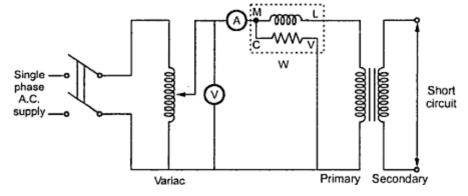Fig. 3 Fig 1. Experimental circuit for O.C. test

The secondary is short circuited with the help of thick copper wire or solid link. As high voltage side is always low current side, it is convenient to connect high voltage side to supply and shorting the low voltage side.
As secondary is shorted, its resistance is very very small and on rated voltage it may draw very large current. Such large current can cause overheating and burning of the transformer. To limit this short circuit current, primary is supplied with low voltage which is just enough to cause rated current to flow through primary which can be observed on an ammeter. The low voltage can be adjusted with the help of variac. Hence this test is also called low voltage test or reduced voltage test. The wattmeter reading as well as voltmeter, ammeter readings are recorded. The observation table is as follows,

Now the current flowing through the windings are rated current hence the total copper loss is full load copper loss. Now the voltage supplied is low which is a small fraction of the rated voltage. The iron losses are function of applied voltage. So the iron losses in reduced voltage test are very small. Hence the wattmeter reading is the power loss which is equal to full load copper losses as iron losses are very low.
...           Wsc = (Pcu) F.L. = Full load copper loss
Calculations : From S.C. test readings we can write,
Wsc = Vsc Isc cos Φsc
...            cos Φsc = Vsc Isc /Wsc = short circuit power factor
Wsc = Isc2 R1e = copper loss
...             R1e =Wsc /Isc2
while        Z1e =Vsc /Isc = √(R1e2 + X1e2)
...             X1e = √(Z1e2 - R1e2)
Thus we get the equivalent circuit parameters R1e, X1e and Z1e. Knowing the transformation ratio K, the equivalent circuit parameters referred to secondary also can be obtained.
Important Note : If the transformer is step up transformer, its primary is L.V. while secondary is H.V. winding. In S.C. test, supply is given to H.V. winding and L.V is shorted. In such case we connect meters on H.V. side which is transformer secondary through for S.C. test purpose H.V side acts as primary. In such case the parameters calculated from S.C. test readings are referred to secondary which are R2e, Z2e and X2e. So before doing calculations it is necessary to find out where the readings are recorded on transformer primary or secondary and accordingly the parameters are to be determined. In step down transformer, primary is high voltage itself to which supply is given in S.C. test. So in such case test results give us parameters referred to primary i.e. R1e, Z1e and X1e.
Key point : In short, if meters are connected to primary of transformer in S.C. test, calculations give us R1e and Z1e if meters are connected to secondary of transformer in S.C. test calculations give us R2e and Z2e.
1.3 Calculation of Efficiency from O.C. and S.C. Tests
We know that,
From O.C. test, Wo =  Pi
From S.C. test,  Wsc = (Pcu) F.L.

Thus for any p.f. cos Φ2 the efficiency can be predetermined. Similarly at any load which is fraction of full load then also efficiency can be predetermined as,

where       n = fraction of full load

where       I2= n (I2) F.L.
1.4 Calculation of Regulation
From S.C. test we get the equivalent circuit parameters referred to primary or secondary.
The rated voltages V1, V2 and rated currents (I1) F.L. and (I2) F.L. are known for the given transformer. Hence the regulation can be determined as,

where I1, I2 are rated currents for full load regulation.
For any other load the currents I1, I2 must be changed by fraction n.
...    I1, I2 at any other load = n (I1) F.L., n (I2) F.L.
Key Point : Thus regulation at any load and any power factor can be predetermined, without actually loading the transformer.

Example 1 : A 5 KVA, 500/250 V, 50 Hz, single phase transformer gave the following readings,
O.C. Test : 500 V, 1 A, 50 W (L.V. side open)
S.C. Test : 25 V, 10 A, 60 W (L.V. side shorted)
Determine : i) The efficiency on full load, 0.8 lagging p.f.
ii) The voltage regulation on full load, 0.8 leading p.f.
iii) The efficiency on 60% of full load, 0.8 leading p.f.
iv) Draw the equivalent circuit referred to primary and insert all the values in it.
Solution : In both the tests, meters are on H.V. side which is primary of the transformer. Hence the parameters obtained from test results will be referred to primary.
From O.C. test,      Vo = 500 V,    Io = 1 A,     Wo= 50 W
...                         cos Φo = Wo/Vo Io = 50/(500x1) = 0.1
...                          Ic = Io cos = 1 x 0.1 = 0.1 A
and                       Im = Io sin Φo = 1 x 0.9949 = 0.9949 A
...                           Ro =Vo /Ic = 500/0.1 = 5000 Ω
and                        Xo = Vo/Im = 500/0.9949 = 502.52 Ω
and                        Wo = Pi= iron losses = 50 W
From S.C. test,      Vsc = 25 V, Isc = 10 A, Wsc = 60 W
...                           R1e = Wsc /Isc2 = 60/(10)2 = 0.6 Ω
Z1e = Vsc /Isc = 25/10 = 2.5 Ω
...                           X1e= √(2.52 - 0.62) = 2.4269 Ω
(I1) F.L. = VA rating/V1
= (5 x 103 )/500 = 10 A
and                           Isc = (I1) F.L.
...                             Wsc = (Pcu) F.L. = 60 W
i) η  on full load, cos = 0.8 lagging

ii) Regulation on full load, cos Φ2 = 0.8 leading

= - 1.95 %
iii) For 60% of full load, n = 0.6 and cos Φ2 = 0.8 leading]
...                   Pcu = copper loss on new load = n2 x (Pcu) F.L.
= (0.6)2 x 60 = 21.6 W
= 97.103 %
iv) The equivalent circuit referred to primary is shown in the Fig. 4.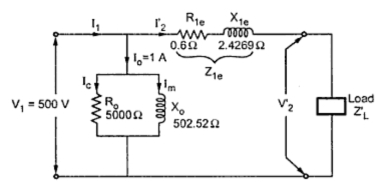Fig. 4

Example 2 : The open circuit and short circuit tests on a 10 KVA, 125/250 V, 50 Hz, single phase transformer gave the following results :
O.C. test : 125 V, 0.6 A, 50 W (on L.V. side)
S.C. test : 15 V, 30 A. 100 W (on H.V. side)
Calculate : i) copper loss on full load
ii) full load efficiency at 0.8 leading p.f.
iii) half load efficiency at 0.8 leading p.f.
iv) regulation at full load, 0.9 leading p.f.
Solution : From O.C. test we can weite,
Wo = Pi = 50 W = Iron loss
From S.C. test we can find the parameters of equivalent circuit. Now S.C. test is conducted on H.V. side i.e. meters are on H.V. side which is transformer secondary. Hence parameters from S.C. test results will be referred to secondary.
Vsc = 15 V, Isc = 30 A, Wsc = 100 W
...            R2e = Wsc/(Isc)2 =10/(30)2 = 0.111Ω
Z1e = Vsc /Isc = 15/30 = 0.5 Ω
...            X2e = √(Z2e2 - R2e2) = 0.4875 Ω
i) Copper loss on full load
(I2) F.L. = VA rating/V2 = (10 x 103)/250 = 40 A
In short circuit test, Isc = 30 A and not equal to full load value 40 A.
Hence Wsc does not give copper loss on full load
...              Wsc = Pcu  at 30 A = 100 W
Now          Pcu α I2
( Pcu at 30 A)/( Pcu at 40 A) = (30/40) 2
100/( Pcu at 40 A) = 900/1600
Pcu at 40 A = 177.78 W
...                (Pcu) F.L. = 177.78 W
ii) Full load η , cos Φ2 = 0.8

iii) Half load η , cos Φ2 = 0.8
n = 0.5 as half load,            (I2) H.L. = 0.5 x 40 = 20= 97.69%
iv) Regulation at full load, cos Φ = 0.9 leading

= -1.8015%

Related articles :
Sponsored links :

#### 10 comments:

1.Perfect post for beginners...!!!
10/10

2.I Really like this infomation.This is a very perfect post.Please visit my Iwebsite.........transformer testing

3.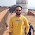This comment has been removed by the author.

4.Perfect information for beginners on oc and sc test on single phase transformers thank you

5.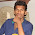powerfactor diagram is wrong

6.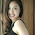The simple truth is I like this article, the author's writing was very honest, very clever writing skills.

Nersha
www.gofastek.com

7.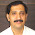Transformers need to be tested in various ways to ensure proper functioning and protection against hazards. There are a number of testing procedures to be followed for confirming the specifications and performance of electrical transformers.
Power Transformers in India | Wire Harness Manufacturer in India

8.Damn! He is great.

9.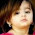It's very perfect post

10.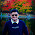i have a doubt.......u cannot measure short ckt. voltage at secondary side ...hence you measure it from voltmeter by primary side and then you put values of short ckt. voltage and current [measured at short ckt. side] to find other parameters...it should be incorrect.